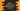# Find the product of all odd, even numbers in a python list## Introduction :

In this tutorial, we will learn how to find the product of all odd and even numbers in a list. The program will take the list values as input from the user and print out the product. With this program, you will learn how to use a for loop,if-else condition and how to take inputs in python.

## Python program :

Python program to find the product of all odd and even numbers in an user-provided list :

``````#1
my_list = []

#2
total = int(input("How many numbers you want to add to the list : "))

#3
for i in range(0, total):
my_list.append(int(input("Enter : ")))

print("You have entered: ", my_list)

#4
odd_product = 1
even_product = 1

#5
for i in my_list:
if(i % 2 == 0):
even_product *= i
else:
odd_product *= i

#6
print("Product of all odd numbers: ", odd_product)
print("Product of all even numbers: ", even_product)``````

### Explanation :

The commented numbers in the above program denote the step numbers below :

1. Create one empty list mylist_.
2. Ask the user to enter the total count of numbers to add to the list. Read and store it in total variable.
3. Run one for loop and read each element as an input from the user. Print the list to the user.
4. Create two variables to hold the product of all odd and even numbers.
5. Iterate through each element of mylist_ using a for-in loop. For each element, check if the current number is even or odd. If it is even, multiply it with evenproduct. Else, multiply it with _oddproduct_.
6. Finally, print out the products.

### Sample Output :

``````How many numbers you want to add to the list : 5
Enter : 2
Enter : 4
Enter : 9
Enter : 10
Enter : 5
You have entered:  [2, 4, 9, 10, 5]
Product of all odd numbers:  45
Product of all even numbers:  80``````